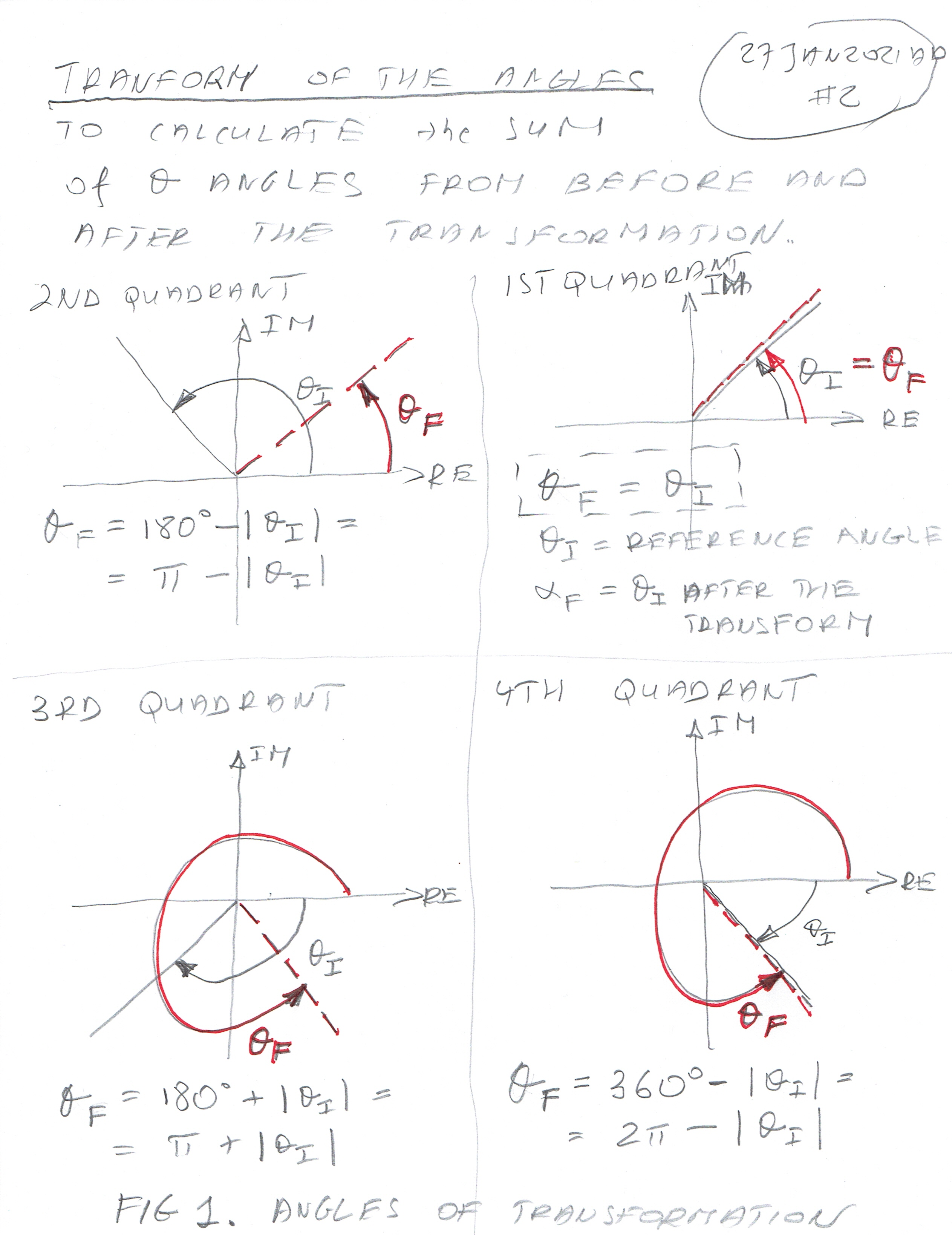9 February 2021 AD

St Cyril of Alexandria (444); St Apollonia (249); St Nicephorus (260)

In this short but necessary article, I will explain the Transformation of the Mixing (Oscillation) Angles in the complex plane.

This is second of three introductory articles before I go into more complicated things. I hope it will make understanding of Math and Quantum involved a bit easier.

On the attached graph you can see clearly the transform of the angles into the 1st and 4th quadrants, i.e. a positive part of the Real Plane. (positive RE-axis).

Basically, a lot was said about it in the following article  99. The narrative for the article "100. Transform of Mixing Angles"

If you prefer to read it as a text - the description is here:

The transformation:

The Transformation computes "Final Angle" - this is a set of rules to get all the angles Θ from previous calculations and transform them into a set of angles with the following values:

0.0 deg < ΘFINAL < 90.0 deg and

270.0 deg < ΘFINAL < 360.0 deg

This is how it is done:

1: If the original angle Θ is located in the first quadrant: ΘFINAL = Θ

2: If the original angle Θ is located in the second quadrant: ΘFINAL = ( 180 deg = π ) - | Θ |; i.e. 180 deg - absolute value of theta.

3: If the original angle Θ is located in the third quadrant: ΘFINAL = ( 180 deg = π ) + | Θ |; i.e. 180 deg + absolute value of theta.

4: If the original angle Θ is located in the fourth quadrant: ΘFINAL = ( 360 deg = 2π ) - | Θ |; i.e. 360 deg - absolute value of theta.

I think the graph below is self-explanatory - Figure 1. Angles of Transformation.